In the verge of coronavirus pandemic, we are providing FREE access to our entire Online Curriculum to ensure Learning Doesn't STOP!

# Ex.7.1 Q3 Cubes and Cube Roots - NCERT Maths Class 8

Go back to  'Ex.7.1'

## Question

Find the smallest number by which each of the following numbers must be divided to obtain a perfect cube.

(i) $$81$$

(ii) $$128$$

(iii) $$135$$

(iv) $$192$$

(v) $$704$$

Video Solution
Cubes And Cube Roots
Ex 7.1 | Question 3

## Text Solution

What is unknown?

To find the smallest number by which a given number must be divided to obtain a perfect cube.

Reasoning:

A number is a perfect cube only when each factor in the prime factorization is grouped in triples. Using this concept smallest number to be multiplied can be obtained.

(i)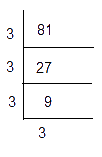\begin{align}81 &= \underline {3 \times 3 \times 3} \times 3\\81 &= {3^3} \times 3\end{align}

Here, the prime factor $$3$$ is not present as triples.

Hence, we divide by $$81$$ by $$3,$$ so that the obtained number becomes a perfect cube.

Thus,

\begin{align}81 \div 3 = 27 = {3^3}\end{align} is a perfect cube.

Hence the smallest number by which $$81$$ should be divided to make a perfect cube is $$3$$.

(ii)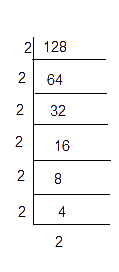\begin{align}128& = \underline {2 \times 2 \times 2} \times \underline {2 \times 2 \times 2} \times 2\\128 &= {2^3} \times {2^3} \times 2\end{align}

Here,the prime factors $$2$$ is not present as triples.

Hence, we divide $$128$$ by $$2,$$ so that the obtained number becomes a perfect cube.

\begin{align}128 \div 2 = 64 = {2^3} \times {2^3} = {4^3}\end{align} is a perfect cube.

Hence the smallest number by which $$128$$ should be divided to make a perfect cube is $$2$$.

(iii)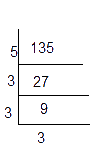\begin{align}135 &= 5 \times \underline {3 \times 3 \times 3} \\135 &= {5^1} \times {3^3}\end{align}

Here, the prime factors $$5$$ is not present as triples.

Hence, we divide $$135$$ by $$5,$$ so that the obtained number becomes a perfect cube.

\begin{align}135 \div 5 = 27 = {3^3}\end{align} is a perfect cube.

Hence the smallest number by which $$135$$ should be divided to make a perfect cube is $$5$$.

(iv)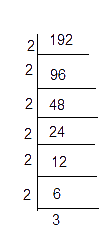\begin{align}192 &= \underline {2 \times 2 \times 2} \times \underline {2 \times 2 \times 2} \times 3\\192 &= {2^3} \times {2^3} \times 3\end{align}

Here,the prime factors $$3$$ is not present as triples.

Hence, we divide $$192$$  by $$3,$$ so that the obtained number becomes a perfect cube.

\begin{align}192 \div 3 = 64 = {2^3} \times {2^3} = {4^3}\end{align} is a perfect cube.

Hence the smallest number by which $$192$$ should be divided to make a perfect cube is $$3$$.

(v)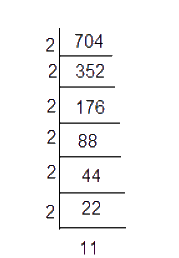\begin{align}704 &= \underline {2 \times 2 \times 2} \times \underline {2 \times 2 \times 2} \times 11\\704 &= {2^3} \times {2^3} \times 11\end{align}

Here, the prime factors $$11$$ is not present as triples.

Hence, we divide $$704$$ by $$11,$$ so that the obtained number becomes a perfect cube.

\begin{align}704 \div 11 = 64 = {2^3} \times {2^3} = {4^3}\end{align} is a perfect cube.

Hence the smallest number by which $$704$$ should be divided to make a perfect cube is $$11$$.

Learn from the best math teachers and top your exams

• Live one on one classroom and doubt clearing
• Practice worksheets in and after class for conceptual clarity
• Personalized curriculum to keep up with school